October 13, 2015

# Example of MyNormProb usage

v = MyNormProb(lb=100,ub=110,mean=100,sd=10,
MyLabel = "Non-Standard Normal RV")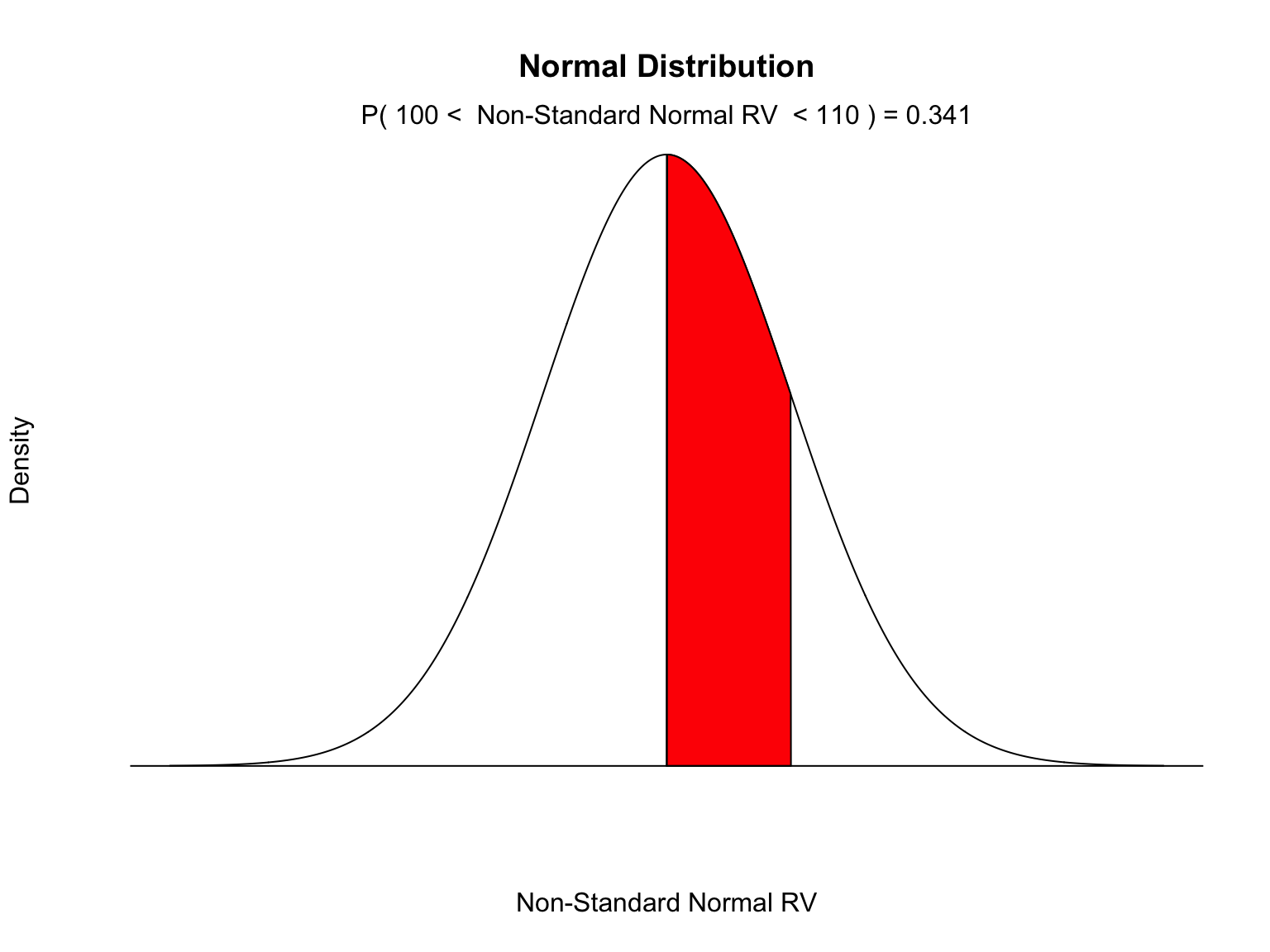v
##  0.3413447

# The Probability Between Two Values Using MyNormProb()

par(mfrow=c(3,1))
MyNormProb(ub=1)
##  0.8413131
MyNormProb(ub = -1)
##  0.1586236
MyNormProb(lb=-1,ub=1)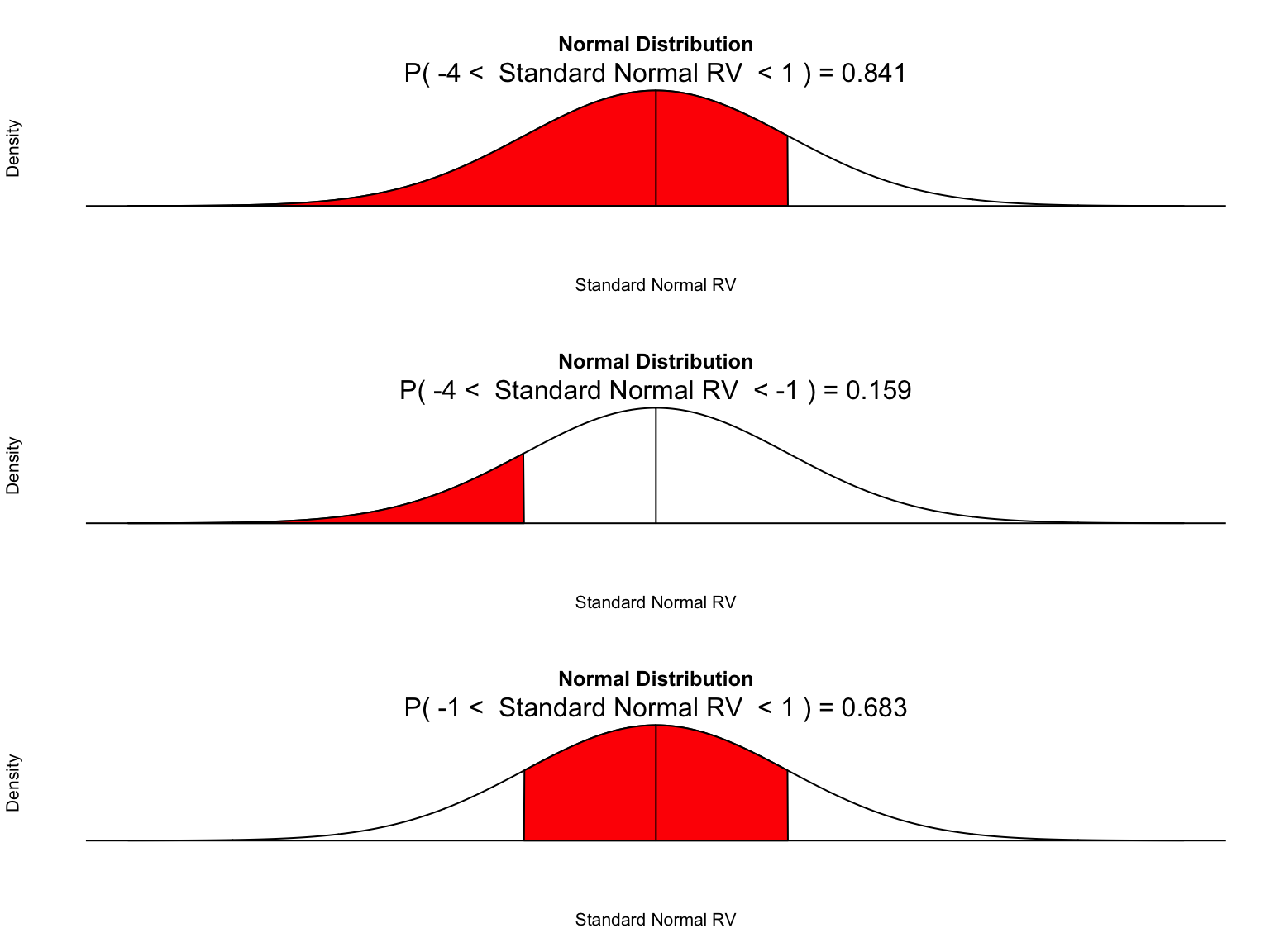##  0.6826895
pnorm(1) - pnorm(-1)
##  0.6826895
par(mfrow=c(1,1))

# Exercise 1

Find the probability that a single random number drawn from a set of numbers with a standard normal distribution is < .7. Do this three ways.

1. Use pnorm()
2. Use MyNormProb() to get a picture.
3. Create a set of 1,000,000 numbers with this distribution and find the fraction that meet the requirement.

# Method 1.
pnorm(.7)
##  0.7580363
# Method 2
MyNormProb(ub=.7)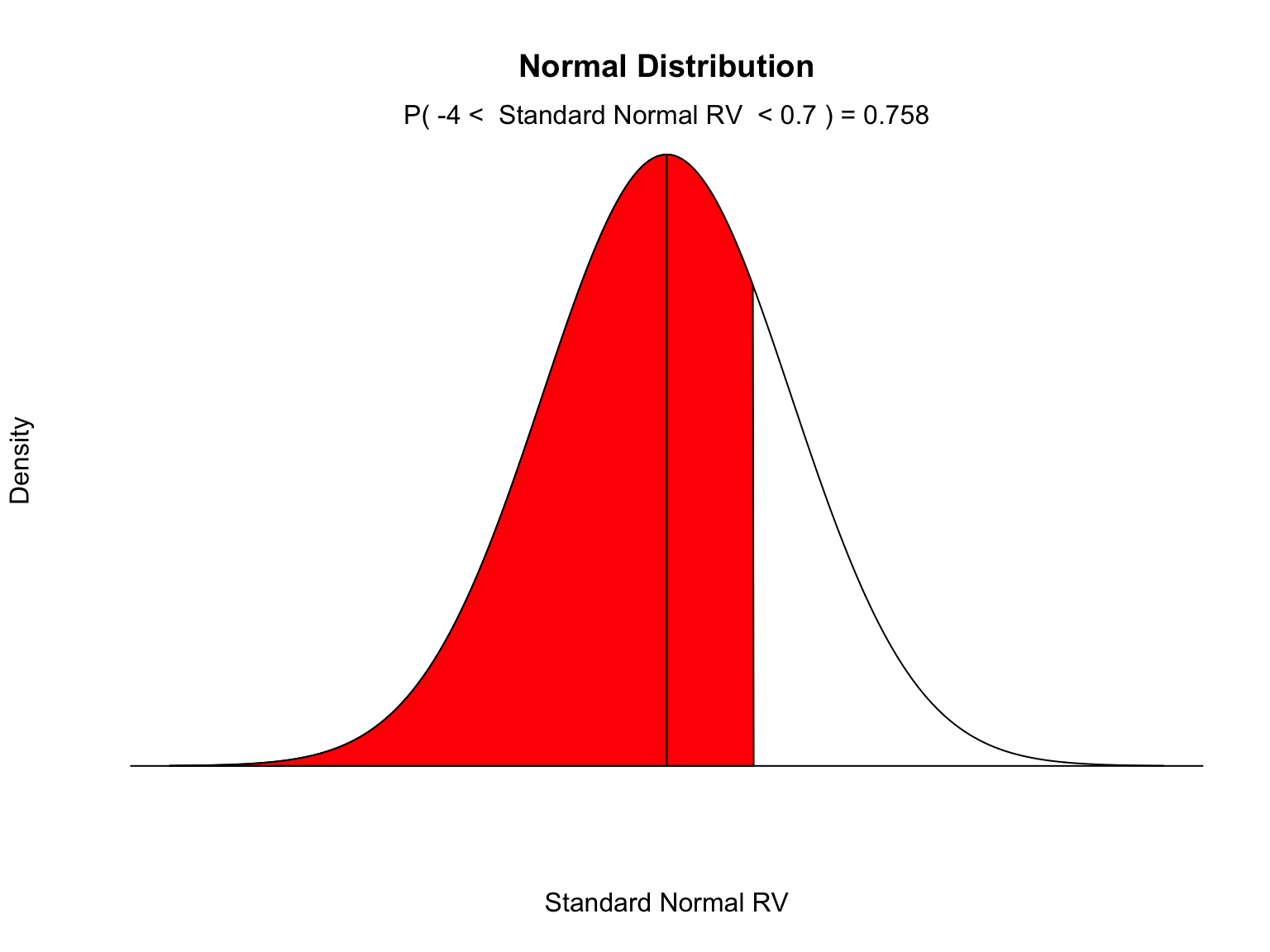##  0.7580047
# Method 3
x = rnorm(1000000)
mean(x<.7)
##  0.758655

# Exercise 2

Find the probability that a single random number drawn from a set of numbers with a standard normal distribution is > .7. Do this three ways.

1. Use pnorm()
2. Use MyNormProb() to get a picture.
3. Create a set of 1,000,000 numbers with this distribution and find the fraction that meet the requirement.

# Method 1
1 - pnorm(.7)
##  0.2419637
# Method 2
MyNormProb(lb=.7)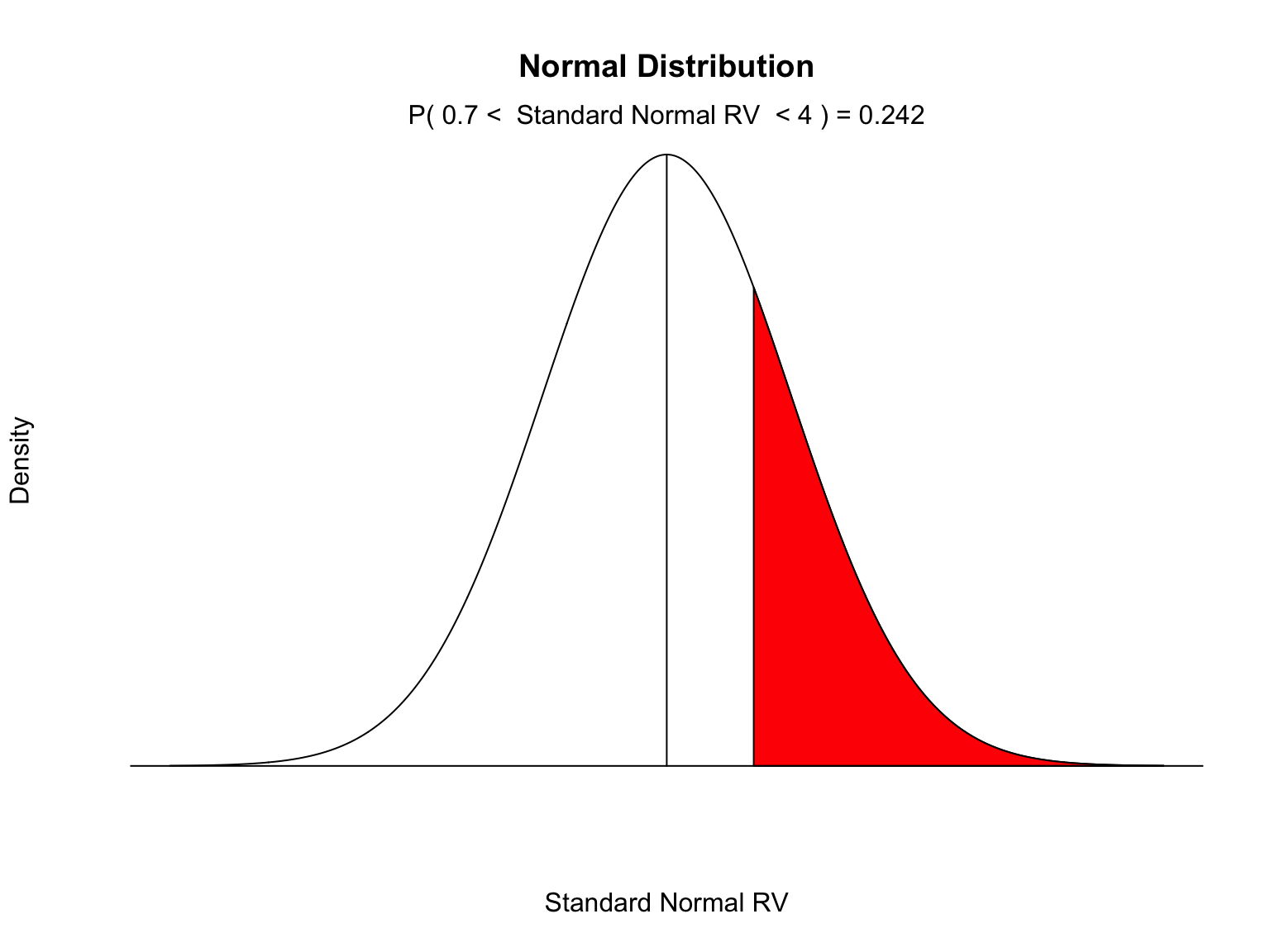##  0.241932
# Method 3
x = rnorm(1000000)
mean(x>.7)
##  0.241482

# Exercise 3

Find the probability that a single random number drawn from a set of numbers with a standard normal distribution is > .3 and < .9. Do this three ways.

1. Use pnorm()
2. Use MyNormProb() to get a picture.
3. Create a set of 1,000,000 numbers with this distribution and find the fraction that meet the requirement.

pnorm(.9) - pnorm(.3)
##  0.1980285
# Method 2
MyNormProb(lb=.3,ub=.9)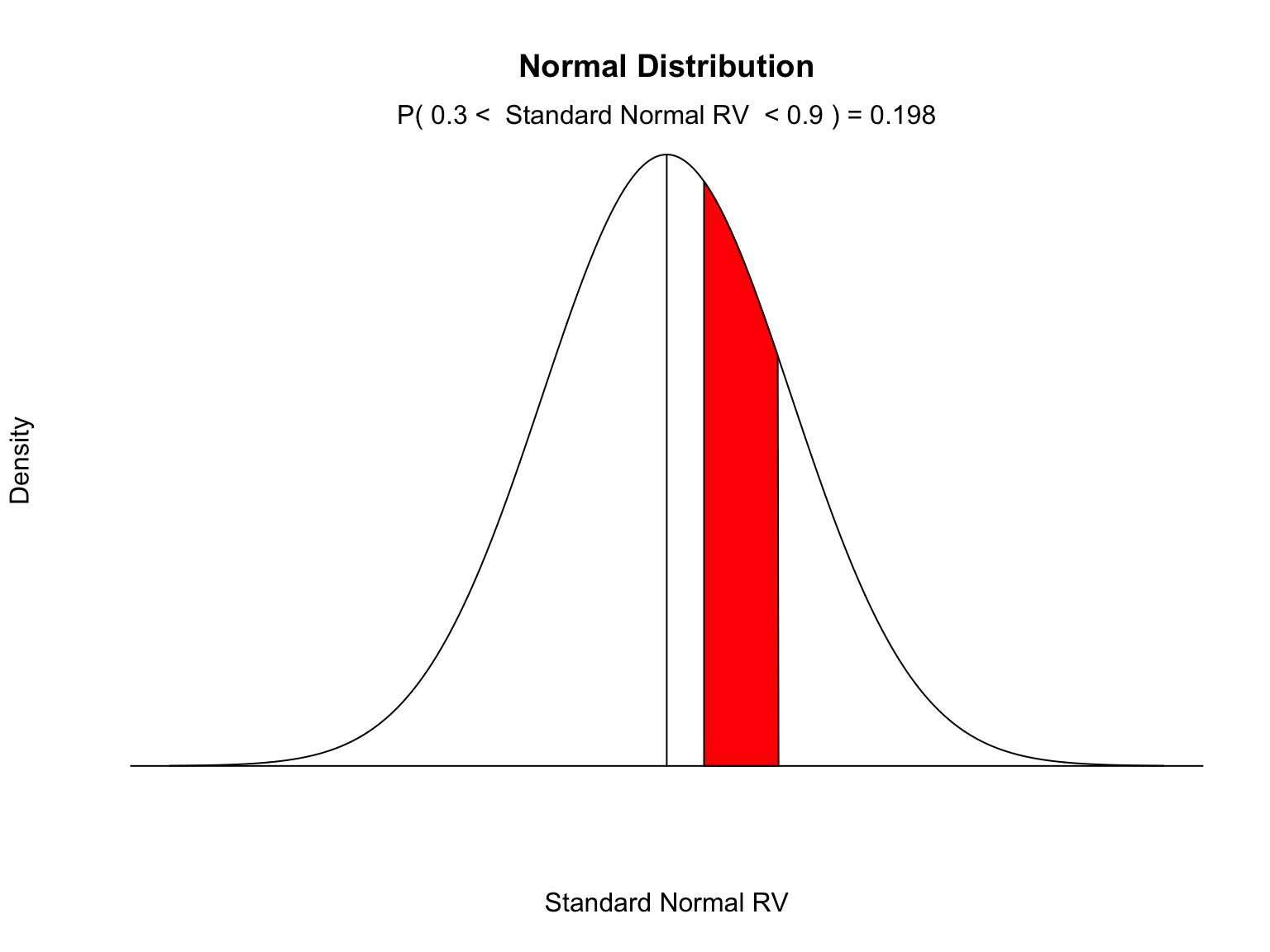##  0.1980285
# Method 3
x = rnorm(1000000)
mean(x>.3 & x < .9)
##  0.198896

# Exercise 4

Find the probability that a single random number drawn from a set of numbers with a normal distribution having a mean of 100 and a standard deviation of 10 is > 90 and < 115. Do this three ways.

1. Use pnorm()
2. Use MyNormProb() to get a picture.
3. Create a set of 1,000,000 numbers with this distribution and find the fraction that meet the requirement.

pnorm(115,mean=100,sd=10) - pnorm(90,mean=100,sd=10)
##  0.7745375
# Method 2
MyNormProb(lb=90,ub=115,mean=100,sd=10,
MyLabel = "Non-Standard Normal RV (100,10)")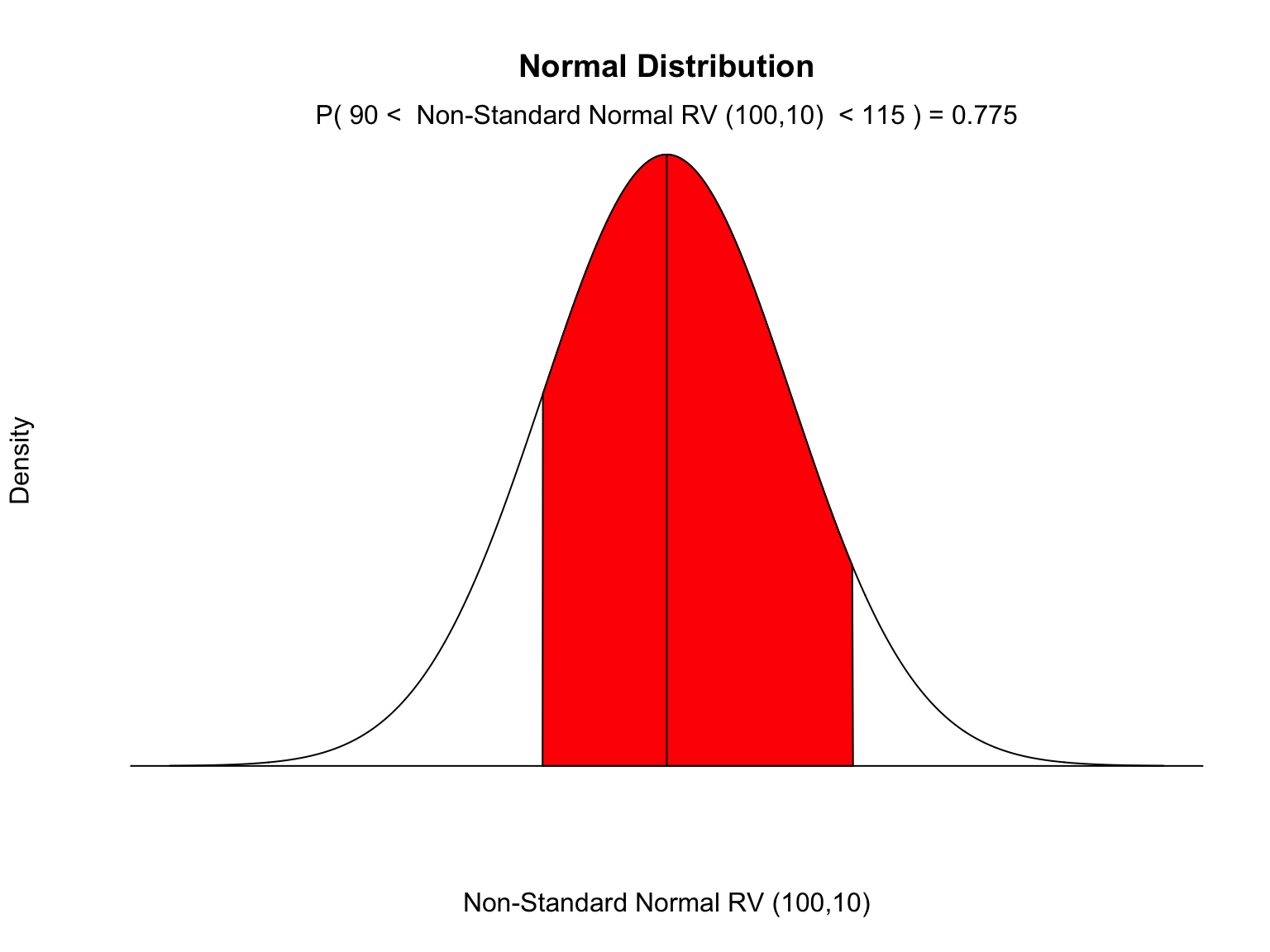##  0.7745375
# Method 3
x = rnorm(1000000,mean=100,sd=10)
mean(x> 90 & x < 115)
##  0.774659

# Exercise 5

90% of a standard normal distribution lies to the left of x. What is the value of x?

qnorm(.9)
##  1.281552

# Exercise 6

10% of a standard normal distribution lies to the right of x. What is the value of x?

qnorm(1 - .1)
##  1.281552

# Exercise 7

A bank’s loan officer rates applicants for credit. The ratings are normally distributed with a mean of 200 and a standard deviation of 50. Find P60, the score which separates the lower 60% from the top 40%.

qnorm(.6,mean=200,sd=50)
##  212.6674

# Exercise 8

The weights of certain machine components are normally distributed with a mean of 8.5 g and a standard deviation of 0.09 g. Find the two weights that separate the top 3% and the bottom 3%. These weights could serve as limits used to identify which components should be rejected.

Top = qnorm(1-.03,mean = 8.5, sd = .09)
Top
##  8.669271
Bottom = qnorm(.03,mean = 8.5, sd = .09)
Bottom
##  8.330729

# Exercise 9

The weights of certain machine components are normally distributed with a mean of 8.5 g and a standard deviation of 0.09 g. What is the probability that a randomly selected component weighs more than 8.6 g.

1 - pnorm(8.6,mean=8.5,sd=.09)
##  0.1332603